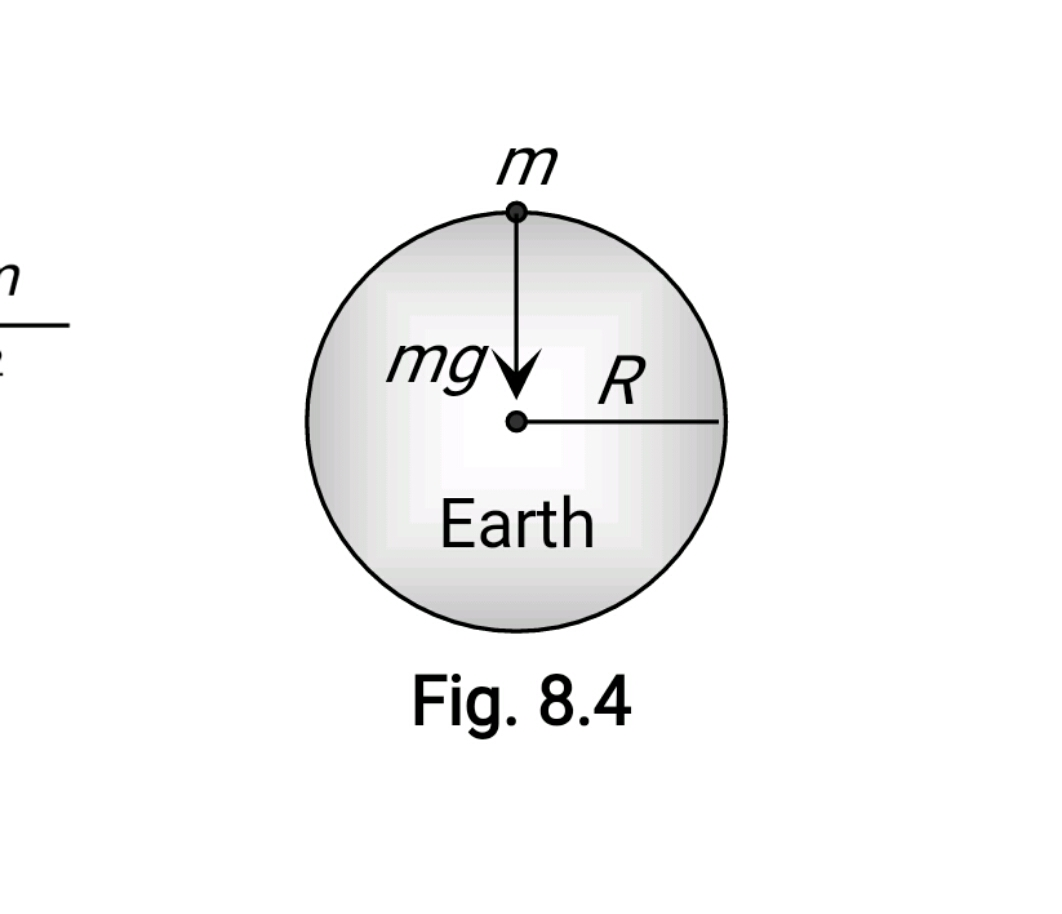#### If the acceleration due to gravity of a planet is half the acceleration due to gravity of earth’s surface and radius of planet is half the radius of the earth, the mass of planet in terms of mass of earth is Option 1)  Me/2  Option 2) Me/4 Option 3) Me/6 Option 4) Me/8As we learnt in

Acceleration due to gravity (g) -

Force extended by earth on a body is gravity.

Formula:gravitydensity of earthRadius of earth

- wherein

It's average value ison the surface of earthandOption 1)This is incorrect option

Option 2)This is incorrect option

Option 3)This is incorrect option

Option 4)This is correct option